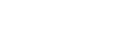# Problem: How many electrons can have the following quantum sets? a. In the 7 th shell of an atom (n = 7)b. n = 5, ℓ = 2c. n = 6, ℓ = 3,  m ℓ = -2d. n = 4, ℓ = 2,  m ℓ = 0 , m s = -1/2e. n = 4, m ℓ = -1f. n = 5, m ℓ = 0 , m s = 1/2g. n = 9, ℓ = 4, m s = -1/2h. n = 2, m s = 1/2

###### FREE Expert Solution
96% (260 ratings)
###### Problem Details

How many electrons can have the following quantum sets?

a. In the 7 th shell of an atom (n = 7)

b. n = 5, ℓ = 2

c. n = 6, ℓ = 3,  m  = -2

d. n = 4, ℓ = 2,  m  = 0 , m s = -1/2

e. n = 4, m  = -1

f. n = 5, m  = 0 , m s = 1/2

g. n = 9, ℓ = 4, m s = -1/2

h. n = 2, m s = 1/2What scientific concept do you need to know in order to solve this problem?

Our tutors have indicated that to solve this problem you will need to apply the The Spin Quantum Number concept. You can view video lessons to learn The Spin Quantum Number. Or if you need more The Spin Quantum Number practice, you can also practice The Spin Quantum Number practice problems.

What is the difficulty of this problem?

Our tutors rated the difficulty ofHow many electrons can have the following quantum sets? a. I...as high difficulty.

How long does this problem take to solve?

Our expert Chemistry tutor, Jules took 13 minutes and 5 seconds to solve this problem. You can follow their steps in the video explanation above.

What professor is this problem relevant for?

Based on our data, we think this problem is relevant for Professor Zhang's class at USF.